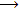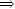# Describe the sequence of a function

Publish On: 2019-03-15

Total Post: 542

# Mjay Jollay

Total Post: 0

## ANS: Describe the sequence of a function

A sequence is a special case of a function whose domain is the set of all natural numbers N and the range is any set, i.e. a function ƒ : NS is called a sequence. If the range of sequence is any subset of real numbers, then the sequence is called a real sequence.

∴ A real sequence is a function from the set N of natural numbers into the set R of real numbers. In this aspect we shall be dealing with real numbers only, therefore, we shall use the term sequence to denote a real sequence.

∴ A sequence is a rule which associates to each natural number a unique real number.

i.e. if ƒ is a sequenceƒ : NR∀ n ϵ N there exist ƒ(n) ϵ R, where ƒ(n) denotes the real number associated by ƒ to n ϵ N.

The range of the sequence is the set of the values

{ƒ(1), ƒ(2), ….. , ƒ(n), … , } ⊂ R.